# Your Solutions - Stage 4 & 5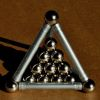### Tetra Inequalities

##### Age 16 to 18Challenge Level

Can you prove that in every tetrahedron there is a vertex where the three edges meeting at that vertex have lengths which could be the sides of a triangle?### A Very Shiny Nose?

##### Age 16 to 18Challenge Level

This problem explores the biology behind Rudolph's glowing red nose, and introduces the real life phenomena of bacterial quorum sensing.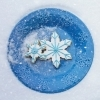### The Koch Snowflake

##### Age 16 to 18Challenge Level

Explore the strange geometrical properties of the Koch Snowflake.### Amicable Arrangements

##### Age 16 to 18Challenge Level

Three of Santa's elves and their best friends are sitting down to a festive feast. Can you find the probability that each elf sits next to their bestie?### Tetra Slice

##### Age 16 to 18Challenge Level

Can you prove that a quadrilateral drawn inside a tetrahedron is a parallelogram?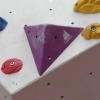### Tetra Perp

##### Age 16 to 18Challenge Level

Show that the edges $AD$ and $BC$ of a tetrahedron $ABCD$ are mutually perpendicular if and only if $AB^2 +CD^2 = AC^2+BD^2$. This problem uses the scalar product of two vectors.### Pythagoras for a Tetrahedron

##### Age 16 to 18Challenge Level

In a right-angled tetrahedron prove that the sum of the squares of the areas of the 3 faces in mutually perpendicular planes equals the square of the area of the sloping face. A generalisation of Pythagoras' Theorem.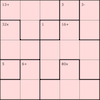### 5 by 5 Mathdokus

##### Age 7 to 16Challenge Level

Can you use the clues to complete these 5 by 5 Mathematical Sudokus?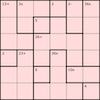### 6 by 6 Mathdokus

##### Age 7 to 16Challenge Level

Can you use the clues to complete these 6 by 6 Mathematical Sudokus?